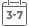# Understanding Biostatistics

• Publish Date: 2011-04-18
• Binding: Hardcover
• Author: Anders Klln
• Sale
• \$215.44
• Regular price \$154.69Understanding Biostatistics looks at the fundamentals of biostatistics, using elementary statistics to explore the nature of statistical tests.

This book is intended to complement first-year statistics and biostatistics textbooks. The main focus here is on ideas, rather than on methodological details. Basic concepts are illustrated with representations from history, followed by technical discussions on what different statistical methods really mean. Graphics are used extensively throughout the book in order to introduce mathematical formulae in an accessible way.

Key features:

• Discusses confidence intervals and p-values in terms of confidence functions.
• Explains basic statistical methodology represented in terms of graphics rather than mathematical formulae, whilst highlighting the mathematical basis of biostatistics.
• Looks at problems of estimating parameters in statistical models and looks at the similarities between different models.
• Provides an extensive discussion on the position of statistics within the medical scientific process.
• Discusses distribution functions, including the Guassian distribution and its importance in biostatistics.

This book will be useful for biostatisticians with little mathematical background as well as those who want to understand the connections in biostatistics and mathematical issues.

MORE FROM THIS COLLECTION